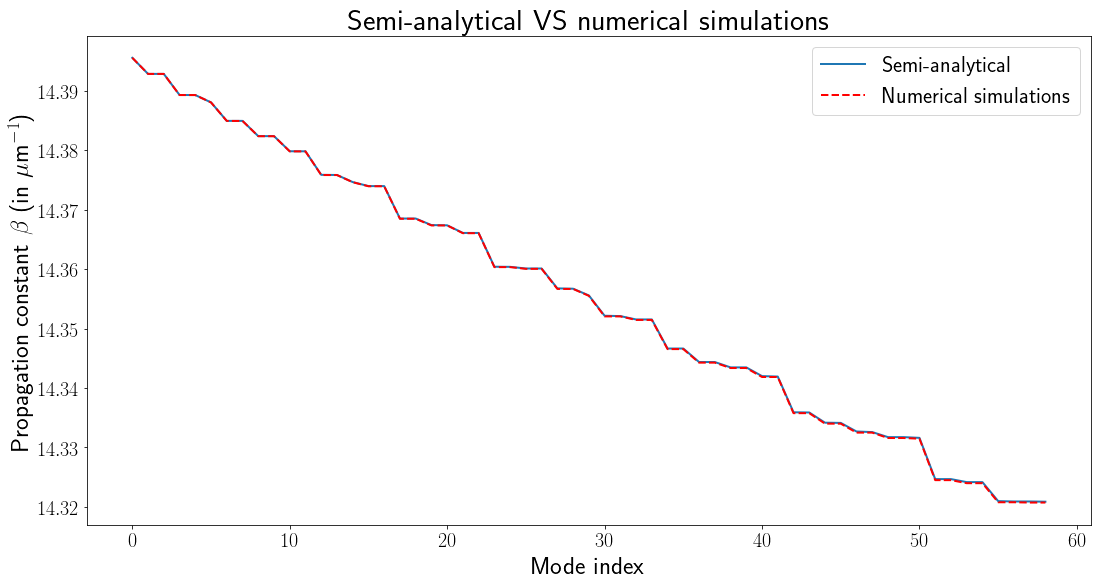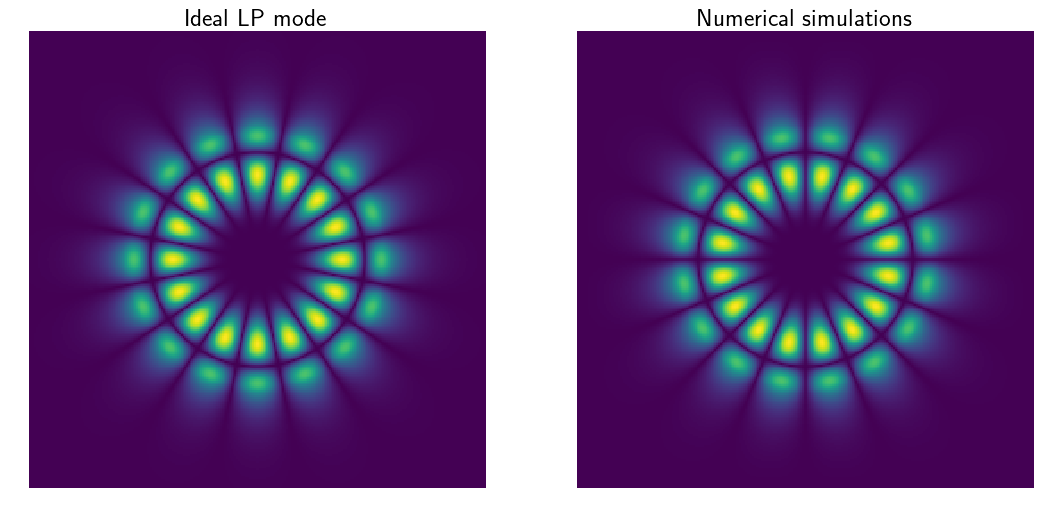## Part 1: Step Index Benchmark

I recently published a two-part tutorial on how to find the modes of an arbitrary multimode fiber without or with bending. Based on this tutorial, I published a (still experimental) version of a Python module to find the modes of multimode fibers and calculate their transmission matrix: pyMMF. The goal of this module is not to compete with commercial solutions in terms of precision but to provide a way to easily simulate realistic fiber systems. To validate the approach, I use step-index multimode fibers as a benchmark test as the dispersion relation is analytically known (see my tutorial here) and for which the Linearly Polarized (LP) mode approximation yields good results. I focus my attention here on the precision of the numerically found propagation constants.

## Preamble

The module and the example I present here work for Python 3 and use standard scientific modules, i.e. numpy, scipy and matplotlib. If you find this module useful for your work, please consider citing it using the following DOI:The up to date version of the code is available here:

https://github.com/wavefrontshaping/pyMMF

The full code of the example presented below is available as a Jupyter Notebook file on my GitHub account:

benchmark_step_index.ipynb

## Setting and Initialization of the Solver

First import the module

import pyMMF

Then define the parameters of the fiber

## Parameters
NA = 0.15
radius = 10 # in microns
n1 = 1.45
wl = 0.6328 # wavelength in microns

We then need to define the parameters of the numerical solver, i.e. the size of the window we will perform calculations on and the number of points in the discretization grid.

areaSize = 3.5*radius # calculate the field on an area larger than the diameter of the fiber
npoints = 2**8 # resolution of the window

As for any discretized based simulation, the solution ideally converges to the true solution when these parameters tend to infinity. One of the goals of the benchmark test is to estimate what these values need to be set to estimate correctly of the propagating modes. Intuitively, a window too small will impact the higher-order mode for which the exponential decrease of the field in the cladding is the slowest. However, for a fixed number of points, increasing the window size will increase the discretization length of the space, yielding inaccurate results for the modes with high spatial frequencies, i.e. the highest order modes again. A trade-off has to be found to give the best results for reasonable calculation times.

We then initialize the solver:

# Create the fiber object
profile = pyMMF.IndexProfile(npoints = npoints, areaSize = areaSize)
# Initialize the index profile
# Instantiate the solver
solver = pyMMF.propagationModeSolver()
# Set the profile to the solver
solver.setIndexProfile(profile)
# Set the wavelength
solver.setWL(wl)

The core of the code is to find the modes by numerically finding the eigenvalues and eigenvectors of a matrix representing the propagation operator in the discretized space (see the tutorial here). This matrix is huge, of size npoints**4, but very sparse. The code does not invert the matrix but finds a given number of eigenvalues/eigenvectors. We then have to tell him how many eigenvalues it should compute. To do so, we first estimate the number of modes using the V number (or normalized frequency parameter, more information here). This function is implemented in pyMMF as estimateNumModesSI().

# Estimate the number of modes for a graded index fiber
Nmodes_estim = pyMMF.estimateNumModesSI(wl,radius,NA,pola=1)

## Calculation of the modes

Semi-analytical solution

As ground truth, we first find the modes using analytical dispersion relation of the modes for step-index fibers. The mode profiles are approximated with LP modes. The Mode object returned contains all the information about the modes.

modes_semianalytical = solver.solve(mode = 'SI', curvature = None)

Numerical calculations

As stated above, we need to tell the program the maximum number of modes it should compute. The program actually computes as many eigenvalues but returns only the ones corresponding to propagating modes if propag_only is set to True. nmodesMax is set to a bit more than the estimation found using the V number to be safe.

modes_eig = solver.solve(nmodesMax=Nmodes_estim+10,boundary = 'close', mode = 'eig', curvature = None, propag_only=True)

## Comparison of the results

We compare the propagation modes found using the semi-analytical method $$\beta_{SA}^i$$ and the ones found with the numerical simulation $$\beta_{num}^i$$. First of all, good news, the numerical method finds the same number of modes, 59 for the parameters we set.We then calculate the average and maximum absolute error divided by the range of propagation constants to estimate the accuracy results. For this set of parameters, we find:

$$\frac{\left\langle\left|\beta_{num}-\beta_{SA}\right|\right\rangle}{\beta_{max}-\beta_{min}} = 1.06\,\,10^{-3}$$

$$\frac{\max{}\left(\left|\beta_{num}-\beta_{SA}\right|\right)}{\beta_{max}-\beta_{min}}=2.54\,\,10^{-3}$$

We then compare qualitatively the mode profile by displaying the mode with the lowest propagation constant:Note that because there are degenerate modes, the numerical solver can find different superpositions of degenerate modes. Mode profiles would look different but would still be accurate.

## Effect of grid parameters

We study here the effect of the number of pixels and the observation window size on the maximum and average error made on the propagation constants as defined previously. We take different values of windows size above 2.4 times the radius of the fiber as for smaller values the code fails to find the correct number of modes.

#### For npoints = 256

$$\frac{\left\langle\left|\beta_{num}-\beta_{SA}\right|\right\rangle}{\beta_{max}-\beta_{min}}$$

$$7.69\,\,10^{-4}$$ $$5.10\,\,10^{-4}$$  $$7.86\,\,10^{-4}$$   $$1.03\,\,10^{-3}$$
$$\frac{\max{}\left|\beta_{num}-\beta_{SA}\right|}{\beta_{max}-\beta_{min}}$$  $$8.13\,\,10^{-3}$$ $$4.89\,\,10^{-3}$$  $$2.12\,\,10^{-3}$$

$$2.54\,\,10^{-3}$$

#### For npoints = 128

$$\frac{\left\langle\left|\beta_{num}-\beta_{SA}\right|\right\rangle}{\beta_{max}-\beta_{min}}$$

$$1.62\,\,10^{-3}$$ $$1.87\,\,10^{-3}$$  $$1.67\,\,10^{-3}$$   $$3.95\,\,10^{-3}$$
$$\frac{\max{}\left|\beta_{num}-\beta_{SA}\right|}{\beta_{max}-\beta_{min}}$$  $$5.23\,\,10^{-3}$$ $$4.98\,\,10^{-3}$$  $$5.65\,\,10^{-3}$$

$$1.07\,\,10^{-3}$$

We note that the optimal parameters for the average error and the maximal one are not the same. Maximal error is always on the highest order modes, which are more sensitive to discretization due to higher spatial frequencies and to boundary conditions due to a slower decay in the cladding.

## Conclusion

Optimal parameters to obtain accurate results depend on the fiber properties. Performing a test on a step-index fiber with a similar core size and numerical aperture is a good idea before performing actual simulations for other types of fibers or when introducing bending. For reasonable calculation times (20 seconds to 2 minutes for the sets of parameters used here), we obtain results where the error made on the propagation constant is of the order of $$10^{-3}\times(\beta_{max}-\beta_{min})$$ which is of the order of $$10^{-4} \mu m^{-1}$$.

See the full IPython code here: benchmark_step_index.ipynb.

Created by sebastien.popoff on 25/02/2019